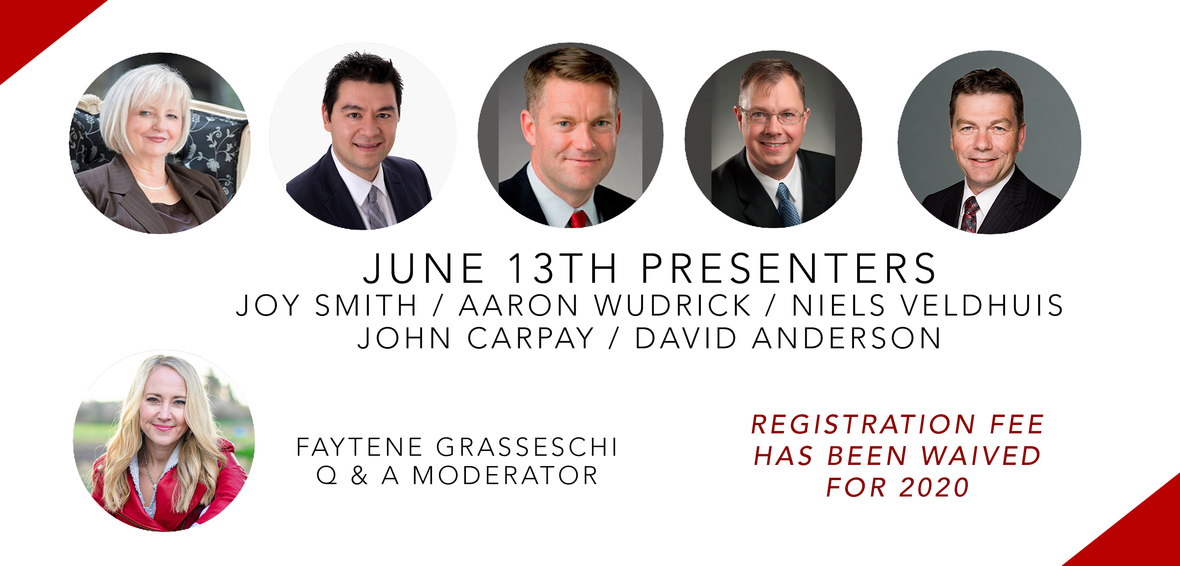Like   Tweet   Pin   +1   in
 /* styles */ June 10, 2020,

On May 25th, George Floyd, a handcuffed black man, died after a white police officer pressed his knee into his neck for 8 minutes and 46 seconds.

All of our hearts have been breaking since seeing the footage the murder. Since this day people worldwide are responding in force to the injustice of racism in 2020.

As you know, in response to the footage protests erupt across Canada. Thousands of people, including politicians such as PM Justin Trudeau joined in the protests. More Info: Global News

All 4 officers involved in this incident have been charged. More Info: CTV News

## TORONTO CHIEF OF POLICE RESIGNS

Last Friday, Toronto Police Chief Mark Saunders took a solidarity knee in downtown Toronto alongside other officers and protesters. And then, on Monday, he stepped down from his role after 37 years of policing service. More info: CBC News

## CPC LEADERSHIP CANDIDATES CHALLENGES PRIME MINISTER JUSTIN TRUDEAU

While millions of Canadians are standing in solidarity against racism many are also questioning why the riots were allowed to proceed while most of the nation is still in lock down due to COVID-19.

CPC Leadership Candidate Leslyn Lewis has written the PM Justin Trudeau addressing these concerns. View this letter here: READ NOW

Please continue to pray for our nation at this volatile time. Let's believe for the healing our nation needs.

Please see some specific prayer points below.

 table div table+table+table+table div table{width:100%;padding:0}table div table+table+table+table div table img{width:96.23%;padding:0;float:none}table div table+table+table+table div table td{width:100%;padding:0 1.88% 18px}/* styles */## WATCH NOW >>

 table.module-5{width:99.62%;padding:0}table div table+table+table+table+table+table div table{width:99.62%;float:none;margin-left:auto;margin-right:auto;padding:0}table div table+table+table+table+table+table div table a{border:0 none;text-decoration:none}table div table+table+table+table+table+table div table img{width:100%!important;border:0 none;text-decoration:none}table div table+table+table+table+table+table div table td{width:100%;padding:0}/* styles */Faytene on her FB live about this issue.
 table div table+table+table+table+table+table+table+table div table{width:100%;padding:0}table div table+table+table+table+table+table+table+table div table img{width:96.23%;padding:0;float:none}table div table+table+table+table+table+table+table+table div table td{width:100%;padding:0 1.88% 18px}/* styles */# Let's Pray For:

▪ For reconciliation and unity between all people groups in Canada
▪ Our nation's leaders to make wise decisions for the well-being of all Canadians in regards to: equality, health, safety, finances
▪ For hurting Canadians to be comforted and heard
▪ For fear to be silenced and overcome and for hope to arise
 ▪ For reconciliation and unity between all people groups in Canada
 ▪ Our nation's leaders to make wise decisions for the well-being of all Canadians in regards to: equality, health, safety, finances
 ▪ For hurting Canadians to be comforted and heard
 ▪ For fear to be silenced and overcome and for hope to arise
 table div table+table+table+table+table+table+table+table+table+table div table{width:100%;padding:0}table div table+table+table+table+table+table+table+table+table+table div table img{width:96.23%;padding:0;float:none}table div table+table+table+table+table+table+table+table+table+table div table td{width:100%;padding:0 1.88% 18px}/* styles */# Help Us Stand For Canada

 table div table+table+table+table+table+table+table+table+table+table+table+table div table{width:100%;padding:0}table div table+table+table+table+table+table+table+table+table+table+table+table div table img{width:96.23%;padding:0;float:none}table div table+table+table+table+table+table+table+table+table+table+table+table div table td{width:100%;padding:0 1.88% 18px}/* styles */table div table+table+table+table+table+table+table+table+table+table+table+table+table div table,table.module-12{width:48.68%;float:left;padding:0}table div table+table+table+table+table+table+table+table+table+table+table+table+table div table a{border:0 none;text-decoration:none}table div table+table+table+table+table+table+table+table+table+table+table+table+table div table img{width:100%!important;border:0 none;text-decoration:none}table div table+table+table+table+table+table+table+table+table+table+table+table+table div table td{width:100%;padding:0 20px 20px 0}/* styles */ Your generosity helps us keep in the fight for Canada. Thank you. Donations can also be send by e-mail to admin@4mycanada.ca
 table div table+table+table+table+table+table+table+table+table+table+table+table+table+table div table{width:100%;padding:0}table div table+table+table+table+table+table+table+table+table+table+table+table+table+table div table img{width:96.23%;padding:0;float:none}table div table+table+table+table+table+table+table+table+table+table+table+table+table+table div table td{width:100%;padding:0 1.88% 18px}/* styles */## Featured Media Clip

 table div table+table+table+table+table+table+table+table+table+table+table+table+table+table+table+table div table{width:100%;padding:0}table div table+table+table+table+table+table+table+table+table+table+table+table+table+table+table+table div table img{width:96.23%;padding:0;float:none}table div table+table+table+table+table+table+table+table+table+table+table+table+table+table+table+table div table td{width:100%;padding:0 1.88% 18px}/* styles */table.module-16{width:99.62%;padding:0}table div table+table+table+table+table+table+table+table+table+table+table+table+table+table+table+table+table div table{width:99.62%;float:none;margin-left:auto;margin-right:auto;padding:0}table div table+table+table+table+table+table+table+table+table+table+table+table+table+table+table+table+table div table a{border:0 none;text-decoration:none}table div table+table+table+table+table+table+table+table+table+table+table+table+table+table+table+table+table div table img{width:100%!important;border:0 none;text-decoration:none}table div table+table+table+table+table+table+table+table+table+table+table+table+table+table+table+table+table div table td{width:100%;padding:0}/* styles */
 table div table+table+table+table+table+table+table+table+table+table+table+table+table+table+table+table+table+table div table{width:100%;padding:0}table div table+table+table+table+table+table+table+table+table+table+table+table+table+table+table+table+table+table div table img{width:96.23%;padding:0;float:none}table div table+table+table+table+table+table+table+table+table+table+table+table+table+table+table+table+table+table div table td{width:100%;padding:0 1.88% 18px}/* styles */table div table+table+table+table+table+table+table+table+table+table+table+table+table+table+table+table+table+table+table div table,table.module-18{width:32.08%;float:right;padding:0}table div table+table+table+table+table+table+table+table+table+table+table+table+table+table+table+table+table+table+table div table a{border:0 none;text-decoration:none}table div table+table+table+table+table+table+table+table+table+table+table+table+table+table+table+table+table+table+table div table img{width:100%!important;border:0 none;text-decoration:none}table div table+table+table+table+table+table+table+table+table+table+table+table+table+table+table+table+table+table+table div table td{width:100%;padding:0 0 20px 20px}/* styles */ Check out Faytene.tv for episodes on key issues facing our nation. Click here to go there now and view recent episodes.
 table div table+table+table+table+table+table+table+table+table+table+table+table+table+table+table+table+table+table+table+table div table{width:100%;padding:0}table div table+table+table+table+table+table+table+table+table+table+table+table+table+table+table+table+table+table+table+table div table img{width:96.23%;padding:0;float:none}table div table+table+table+table+table+table+table+table+table+table+table+table+table+table+table+table+table+table+table+table div table td{width:100%;padding:0 1.88% 18px}/* styles */## ANNOUNCEMENTS //

 table div table+table+table+table+table+table+table+table+table+table+table+table+table+table+table+table+table+table+table+table+table+table div table{width:100%;padding:0}table div table+table+table+table+table+table+table+table+table+table+table+table+table+table+table+table+table+table+table+table+table+table div table img{width:96.23%;padding:0;float:none}table div table+table+table+table+table+table+table+table+table+table+table+table+table+table+table+table+table+table+table+table+table+table div table td{width:100%;padding:0 1.88% 18px}/* styles */## Canada Summit 2020 Online Happening This Saturday

 table div table+table+table+table+table+table+table+table+table+table+table+table+table+table+table+table+table+table+table+table+table+table+table+table+table div table td,table.module-24{width:100%;padding:0}table div table+table+table+table+table+table+table+table+table+table+table+table+table+table+table+table+table+table+table+table+table+table+table+table+table div table{width:100%;float:none;margin-left:auto;margin-right:auto;padding:0}table div table+table+table+table+table+table+table+table+table+table+table+table+table+table+table+table+table+table+table+table+table+table+table+table+table div table a{border:0 none;text-decoration:none}table div table+table+table+table+table+table+table+table+table+table+table+table+table+table+table+table+table+table+table+table+table+table+table+table+table div table img{width:100%!important;border:0 none;text-decoration:none}/* styles */## Saturday June 13th Presenters:

Aaron Wudrick, Canadian Taxpayers Federation, Federal Director
Neils Veldhuis, Fraser Institute, President
John Carpay, Justice Centre for Constitutional Freedoms, President
David Anderson, Former MP and founder of Free to Believe
Joy Smith, Former MP and Joy Smith Foundation

Then, participants will have the option to break into small groups (online) to innovate solutions.

Many of you have already registered. We are excited to see you next week!

If you have already registered, you will be receiving a briefing e-mail next week with instructions and your links to connect.

## NOT REGISTERED YET?

It is not too late to register.

Because of the importance of the conversations at hand, the event has been made FREE this year.

General presentation capacity is unlimited but registration is required.

Innovation sessions will be capped at 225 participants per topic and registration is required.

 table div table+table+table+table+table+table+table+table+table+table+table+table+table+table+table+table+table+table+table+table+table+table+table+table+table+table+table+table div table{width:100%;padding:0}table div table+table+table+table+table+table+table+table+table+table+table+table+table+table+table+table+table+table+table+table+table+table+table+table+table+table+table+table div table img{width:96.23%;padding:0;float:none}table div table+table+table+table+table+table+table+table+table+table+table+table+table+table+table+table+table+table+table+table+table+table+table+table+table+table+table+table div table td{width:100%;padding:0 1.88% 18px}/* styles */